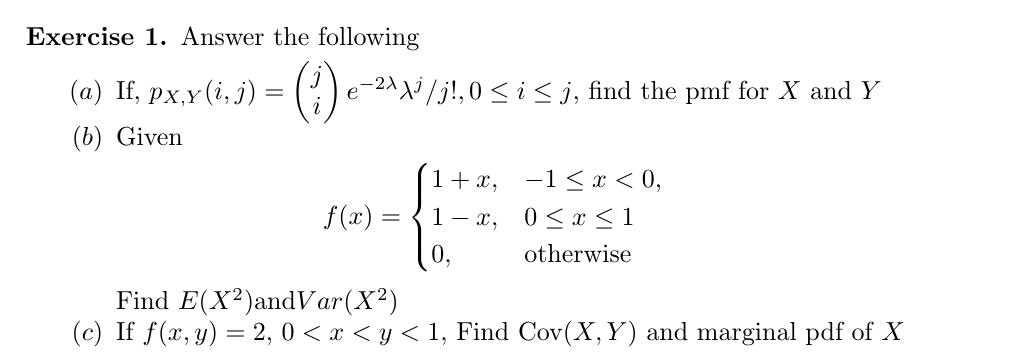# Exercise 1. Answer the following(a) If, px,y (i,) =- 2^^j /j!,0 < i < j, find the pmf for X and Yе(b) Given1 +x-1 x< 0,f(x)< 11 — х, 0

Question
64 views

Please, help me with very detailed and self-explanatory solutions. thank youhelp_outlineImage TranscriptioncloseExercise 1. Answer the following (a) If, px,y (i,) = - 2^^j /j!,0 < i < j, find the pmf for X and Y е (b) Given 1 +x -1 x< 0, f(x) < 1 1 — х, 0<х otherwise Find E(X2)andVar(X2 (c) If f(x, y) 2, 0
check_circle

Step 1

To determine the required probability mass functions

Step 2

(a) pmfs of X and Y are obtained by summing over the Y and X values respectively

Step 3

(b)

...

### Want to see the full answer?

See Solution

#### Want to see this answer and more?

Solutions are written by subject experts who are available 24/7. Questions are typically answered within 1 hour.*

See Solution
*Response times may vary by subject and question.
Tagged in

### Random Variables